# Basic Arithmetic : Order of Operations and PEMDAS

## Example Questions

### Example Question #1 : Order Of Operations And Pemdas

Solve: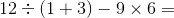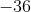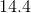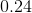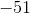Explanation:

Use the order of operations: PEMDAS (Parentheses, Exponents, Multiplication, Division, Addition, Subtraction).

We want to solve what's in the parentheses first.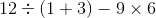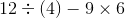Now, do the division and the multiplication.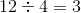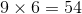Therefore our equation becomes: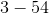Finally, subtract.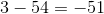### Example Question #1 : Order Of Operations And Pemdas

Solve: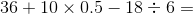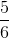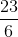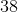Explanation:

Use order of operations, PEMDAS (Parentheses, Exponents, Multiplication, Division, Addition, Subtraction) to solve.

Since there are no parentheses or exponents we can go straight to multiplication and division.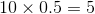and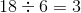Therefore the following happens: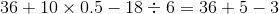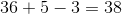### Example Question #1 : Operations In Expressions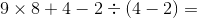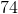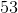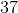Explanation:

Using order of operations, we need to solve whatever is in the parentheses first.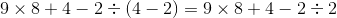Next, do the multiplication and division.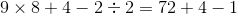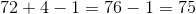### Example Question #1 : Order Of Operations And Pemdas

Simplify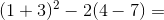Explanation:

We start with what is inside the parentheses, so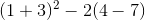becomes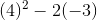.

Next, we take care of any exponents, giving us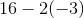.

Next, we take care of multiplication/division, giving us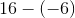or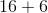.

Finally, we carry out our addition/subtraction, leaving us with.

### Example Question #1 : Order Of Operations And Pemdas

Solve: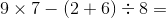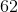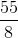Explanation:

Using PEMDAS, we do the parenthetical bit first: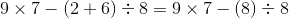Now, we do multiplication and division: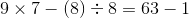Finally, subtract.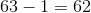### Example Question #2 : Order Of Operations And Pemdas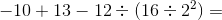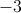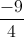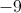Explanation:

This is a classic order of operations question, and if you are not careful, you can end up with the wrong answer!

Remember, the order of operations says that you have to go in the following order of operations: Parentheses, Exponents, Multiplication, Division, Addition, Subtraction (also known as PEMDAS).  In this equation, you will start with the parentheses.  In the parentheses, we have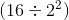.

But within the parentheses, you still need to follow PEMDAS.  First, we will solve the exponent, and the square of 2 is 4.  Then, we'll divide 16 by 4, which gives us 4, so we can rewrite our original equation as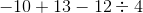.

We can now divideinto, which gives us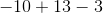The last step is to add and subtract the numbers above, paying careful attention to negative signs.  In the end, we end up withbecause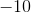added toequals, andminusequals### Example Question #1 : Operations In Expressions

When evaluating the expression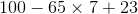,

in which order must you work the three operations?

Explanation:

By the order of operations, in the absence of grouping symbols, multplication must be worked before adding or subtracting. Then the addition and subtraction must be worked in left-to-right order; the subtraction is at left, so the subtraction is worked next, followed by the addition.

### Example Question #1 : Order Of Operations And Pemdas

When evaluating the expression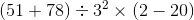,

which of the five operations must be carried out third?

Multiplication

Division

Squaring

Subtraction

Squaring

Explanation:

By the order of operations, any operations within parentheses must be carried out first; there are two here, the addition and the subtraction. After this is done, the exponent, or squaring, must be worked before the other operations. Squaring, the third operation, is therefore the correct answer.

### Example Question #2 : Order Of Operations And Pemdas

When evaluating the expression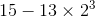,

in which order must you work the three operations?

Cubing, subtraction, multiplication

Subtraction, cubing, multiplication

Multiplication, cubing, subtraction

Subtraction, multiplication, cubing

Cubing, multiplication, subtraction